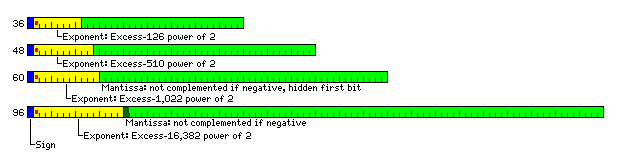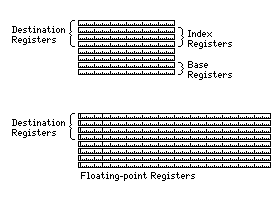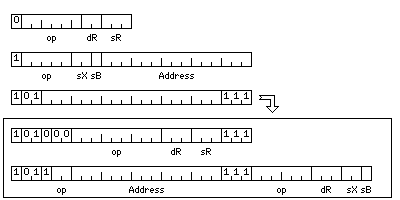[Next][Up/Previous]

# An Illustrative Architecture

Originally, although an example architecture based on a fundamental unit of 12 bits was sketched out on the preceding page, I had declined to explore the possibility in detail. Now I will examine how a useful architecture might be built around instructions that are 12 and 24 bits in length.

The floating-point data formats that it will use would be the following:The extended-precision floating-point numbers would be 96 bits in length, and this would also be a possible width for the data bus in this architecture; thus, some forms of data would face alignment restrictions based on power-of-two multiples of the fundamental unit.

The basic instruction formats would be the following:Addresses, in this architecture, point to consecutive individual 12-bit units of memory.

This is based on organizing the registers of the machine as follows:Thus, in a register-to-register instruction, to allow six bits for the opcode, and a bit to distinguish these instructions from memory-reference instructions, only the first four of the eight registers in each set can be used as the destination.

Note that this means that there will be a register-to-register form of the store instructions.

In the memory-to-register instructions, the destination register will always be register 0. As the base register field is only one bit wide, it indicates if register 6 or register 7 is used as the base register; zero does not turn off the use of a base register, but indicates register 6 instead. The index register field is two bits wide; 0 does indicate that an instruction is not indexed; 1, 2, or 3 indicates the integer register used as the index register.

Given that sizes of 36 bits and 60 bits are used for floating-point numbers, the opportunity for making use of alignment restrictions to conserve opcode space is limited. But as 24-bit and 48-bit integers, as well as 48-bit floating-point numbers, are used, some opportunity does exist, which is why the opcode field of the memory-reference instructions is only five bits long, while that of the register-to-register instructions is six bits long.

The basic register-to-register and memory reference instructons are as follows:

```Register-to-   Memory
Register       Reference

000000         00000 ...    0  SW     Swap
000001         00001 ...    0  C      Compare
000010         00010 ...    0  L      Load
000011         00011 ...    0  ST     Store
000100         00100 ...    0  A      Add
000101         00101 ...    0  S      Subtract
000110         00110 ...    0  M      Multiply
000111         00111 ...    0  D      Divide

001000         01000 ...    0  I      Insert
001001         01001 ...    0  UC     Unsigned Compare
001010         01010 ...    0  UL     Unsigned Load
001011         01011 ...    0  X      XOR
001100         01100 ...    0  N      AND
001101         01101 ...    0  O      OR
001110         01110 ...    0  ME     Multiply Extensibly
001111         01111 ...    0  DE     Divide Extensibly

010000         00000 ...   01  SWL    Swap Long
010001         00001 ...   01  CL     Compare Long
010010         00010 ...   01  LL     Load Long
010011         00011 ...   01  STL    Store Long
010100         00100 ...   01  AL     Add Long
010101         00101 ...   01  SL     Subtract Long
010110         00110 ...   01  ML     Multiply Long
010111         00111 ...   01  DL     Divide Long

011001         01001 ...   01  UCL    Unsigned Compare Long
011010         01010 ...   01  ULL    Unsigned Load Long
011011         01011 ...   01  XL     XOR Long
011100         01100 ...   01  NL     AND Long
011101         01101 ...   01  OL     OR Long
011110         01110 ...   01  MEL    Multiply Extensibly Long
011111         01111 ...   01  DEL    Divide Extensibly Long

100000         00000 ...  011  SWE    Swap Extended
100001         00001 ...  011  CE     Compare Extended
100010         00010 ...  011  LE     Load Extended
100011         00011 ...  011  STE    Store Extended
100100         00100 ...  011  AE     Add Extended
100101         00101 ...  011  SE     Subtract Extended
100110         00110 ...  011  ME     Multiply Extended
100111         00111 ...  011  DE     Divide Extended

101000         01000 ...  011  MEUE   Muliply Extensibly Unnormalized Extended
101001         01001 ...  011  DEUE   Divide Extensibly Unnormalized Extended
101010         01010 ...  011  LUE    Load Unnormalized Extended
101011         01011 ...  011  STUE   Store Unnormalized Extended
101100         01100 ...  011  AUE    Add Unnormalized Extended
101101         01101 ...  011  SUE    Subtract Unnormalized Extended
101110         01110 ...  011  MUE    Multiply Unnormalized Extended
101111         01111 ...  011  DUE    Divide Unnormalized Extended

110000         10000           SWF    Swap Floating
110001         10001           CF     Compare Floating
110011         10011           STF    Store Floating
110101         10101           SF     Subtract Floating
110110         10110           MF     Multiply Floating
110111         10111           DF     Divide Floating

111000         11000           SWD    Swap Double
111001         11001           CD     Compare Double
111011         11011           STD    Store Double
111101         11101           SD     Subtract Double
111110         11110           MD     Multiply Double
111111         11111           DD     Divide Double
```

Because the extended format does not have a hidden first bit, unnormalized operate instructions are available for that format.

For integers, the Load instruction performs sign extension when the register is not filled; the Unsigned Load instruction zeroes the part of the register that is not filled, and the Insert instruction leaves that part of the register unaffected.

The Divide Extensibly instruction treats the destination register as a 48-bit quantity; that is divided by the 24-bit source operand, and the 48-bit result is placed in the destination register. The 24-bit remainder is loaded into the register at the next higher location - and the destination register for this instruction must be an even-numbered register.

The Divide Extensibly Long instruction can only have register zero as its destination register. Registers 0 and 1 together form a 96-bit integer (the most significant part of which is in register 0) which is divided by the 48-bit source operand. The 96-bit quotient is placed in registers 0 and 1, and the 48-bit remainder is placed in register 2 (not register 3, as a strict analogy with the Divide Extensibly instruction might suggest).

The Multiply Extensibly instruction multiplies the 24-bit source operand and the 24-bit destination operand, and puts their 48-bit product in the destination register.

The Multiply Extensibly Long instruction multiplies the 48-bit source operand and the 48-bit destination operand, and puts their 96-bit product in the destination register (which must be an even-numbered register) and the one following, with the most significant part being placed in the destination register.

The Multiply Extensibly Unnormalized Extended instruction places the most significant part of the product of its extended-precision floating-point operands in the destination register, which must be an even-numbered register, and the least-significant part of a double-width mantissa in the register following. The contents of the exponent field in that register are undefined, and should be ignored.

The Divide Extensibly Unnormalized Extended instruction divides a floating-point number with a double-length mantissa spread over two registers by the source operand; a quotient is produced as though the mantissas of the two operands were integers, replacing the source operand, and the remainder from this division is placed, in unnormalized form and with the correct exponent, in the second register following the source register.

The codes that would indicate an instruction operating on an aligned operand 192 bits in length or greater are instead used to permit additional instructions, both register to register and memory-reference.In order to increase the size of the opcode field to eight bits for the memory-reference instructions, it was not possible to increase the size of the index register or base register fields in the instruction; this seemed reasonable, as making additional base registers available just for the extended-format instructions would have been of limited utility. However, a full three-bit destination register field was provided, as is the case for the extended register-to-register instructions.

In the register-to-register instructions, the opcode field is expanded to nine bits, rather than eleven, allowing additional instruction format options.

Seven bits are all that is required for the basic memory-reference and register-to-register instructions. These opcodes follow this pattern:

```000nnnn  (8 bits) Byte
001nnnn (12 bits) Small
010nnnn (24 bits) Integer
011nnnn (36 bits) Alternate Integer
100nnnn (48 bits) Long
101nnnn (96 bits) Extended
1100nnn (36 bits) Floating
1101nnn (48 bits) Medium
1110nnn (60 bits) Double
```

The types before Extended are fixed-point types. Multiply, Divide, Multiply Extensibly, and Divide Extensibly instructions are not provided for the 8 bit long Byte type.

The types after Extended are floating-point types with a hidden first bit, so no unnormalized floating-point instructions are provided for them.

Extended, on the other hand, is a floating-point type without a hidden first bit, so all the instructions shown above are available.

The extended memory-reference instructions are not required to be properly aligned. Alignment is still recommended, however, Thus, for Integer instructions, the last bit of the displacement should be zero; for Long and Medium instructions, the last two bits; and for Extended instructions, the last three bits.

It is envisaged that the data bus to main memory will be at least 96 bits wide, and, if longer, some power-of-two multiple of 96 bits.

To avoid crossing the boundaries of memory words with Floating (or Alternate Integer) numbers or Double numbers, one technique that is available would be to store them at a regular spacing of 96 bits, where each 96-bit memory word would contain one Floating number (36 bits long) and one Double number (60 bits long) in either order. Elaborations of this basic technique for computer architectures with different basic word lengths will be discussed later in this section, on this page.

The basic unit of memory in this machine is 12 bits long. Two of those units are 24 bits long, and three 8-bit bytes will fit into 24 bits. Thus, Byte instructions are regarded as aligned when the last bit of the address field is zero.

Only the first of three bytes in a 24-bit word can be addressed by such a memory-reference instruction in the absence of indexing. Note that a register-to-register Byte instruction operates on the least significant 8 bits of the registers involved, while a memory-reference Byte instruction operates on the byte in memory immediately at the address provided, thus in the most significant 8 bits of the 24-bit quantity addressed. This follows the general pattern of memory-reference instructions, since 12-bit and 24-bit quantities addressed by memory-reference instructions begin with the 12-bit memory cell actually addressed, rather than being in the last 12 bits or the last 24 bits of a 48-bit expanse of memory, in order to imitate the width of an integer register.

When an index register, 1, 2, or 3, is specified to be used with a Byte instruction, instead of providing a normal displacement in units of 12 bits, the displacement is in 8-bit bytes.

However, one byte in every 33 bytes is skipped in order to avoid the need to divide the index register contents by three in order to produce a conventional memory address that points to a 12-bit memory cell, or (after being shifted right three places) a 96-bit memory word.

33 bytes occupy eleven 24-bit words. If only 32 of them are used, then address generation can proceed as follows: the last five bits of the index register contents are used, basically, as an index into a table which indicates which byte of which 24-bit word is to be used, and the remainder of the index register contents are first shifted right five bits, and then multiplied by eleven to obtain a displacement in normal units of 24 bits (which can then be shifted left one bit to obtain a standard address in units of 12-bit memory cells, or shifted right two bits to obtain a physical address in units of 96-bit memory words).

This basic technique, as applied to putting 36-bit and 51-bit floating-point numbers in a 256-bit memory word, or as applied in the general case, will be discussed later in this section on this page.

There is enough opcode space to permit providing for other important types of instruction:The prefixed instructions are designed to minimize the overhead of using types, such as 48-bit floating-point, not provided for in the basic register-to-register or memory-reference instructions; instead of lengthening each instruction by 12 bits, a single 24-bit prefix can provide for a large number of instructions.

Each instruction within the group of instructions so prefixed has a 4-bit opcode, indicating the operation it performs, and one bit to indicate which of two data types, A or B, applies. The first bit of the instruction distinguishes between 12-bit register-to-register instructions and 24-bit memory-reference instructions. The second bit is always zero, except in the last instruction in the sequence of prefixed instructions, indicated by it being a one. Because the normal opcode in a 24-bit memory-reference instruction is only five bits long, the choice of which base register to use for all memory-reference instructions in the sequence of prefixed instructions is made in the prefix.

[Next][Up/Previous]